# Create a banner and buttons with R

with a few lines of code.

1. Load the packages we will need.
``````pacman::p_load(tidyverse, jasmines, magick, here)
``````
1. Creating a background for my banner and buttons for my Canvas course provided the opportunity to try out the jasmines package.

Using the excellent examples as a guide, I created a background by modifying a few lines.

``````entity_lissajous() %>%
unfold_tempest() %>%
style_ribbon(background = "thistle2")
``````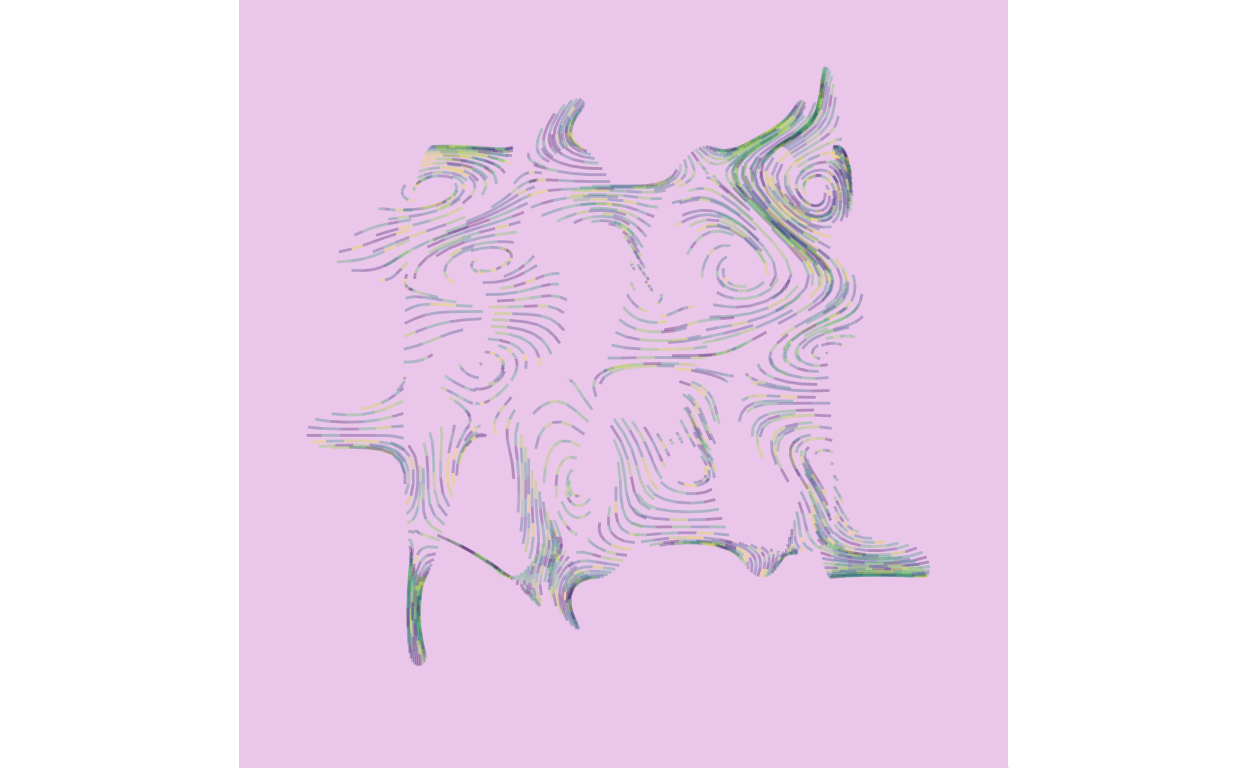1. Save the background to banner.png
``````entity_lissajous() %>%
unfold_tempest() %>%
style_ribbon(background = "thistle2")  %>%
export_image(here(
"_posts", "create-a-banner-and-buttons-with-r", "banner.png"))
``````
1. Read the image back in as a magick object to modify it
``````banner_png  <- image_read(here(
"_posts", "create-a-banner-and-buttons-with-r", "banner.png"))
``````
1. Resize it and add my course name, “Auditing”, to the banner
``````banner_png  %>%
image_resize(
geometry_size_pixels(width = 1000,
height = 200,
preserve_aspect =FALSE ))  %>%
image_annotate("Auditing",
location = geometry_point(x = 350, y = 50),
size = 50, font = "Comic Sans")
``````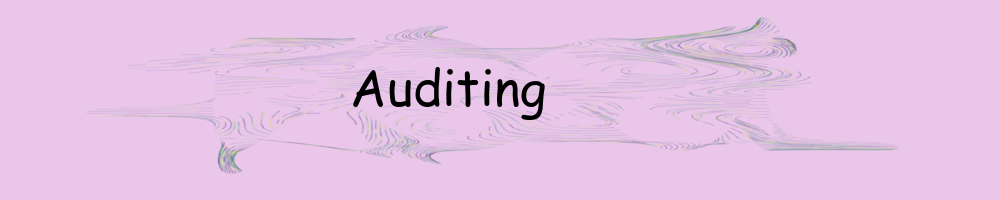1. Save the banner with text as audit_banner.png
``````banner_png  %>%
image_resize(
geometry_size_pixels(width = 1000,
height = 200,
preserve_aspect =FALSE ))  %>%
image_annotate("Auditing",
location = geometry_point(x = 350, y = 50),
size = 50, font = "Comic Sans")  %>%
image_write(
here(
"_posts",
"create-a-banner-and-buttons-with-r","audit_banner.png")
)
``````
``````banner_png  %>%
image_resize(
geometry_size_pixels(width = 150,
height = 75,
preserve_aspect =FALSE )) -> button
button
``````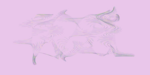``````button  %>% image_annotate("Syllabus",
location = geometry_point(x = 15, y = 10),
size = 30, font = "Comic Sans")  %>%
image_border("black", "4x4")
``````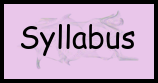``````button   %>% image_annotate("Modules",
location = geometry_point(x = 15, y = 10),
size = 30, font = "Comic Sans")  %>%
image_border("black", "4x4")
``````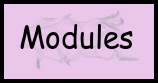1. Write the buttons to png files
``````button  %>% image_annotate("Syllabus",
location = geometry_point(x = 15, y = 10),
size = 30, font = "Comic Sans")  %>%
image_border("black", "4x4")  %>%
image_write(here(
"_posts",
"create-a-banner-and-buttons-with-r","syllabus_btn.png")
)

button   %>% image_annotate("Modules",
location = geometry_point(x = 15, y = 10),
size = 30, font = "Comic Sans")  %>%
image_border("black", "4x4") %>%
image_write(here(
"_posts",
"create-a-banner-and-buttons-with-r","modules_btn.png")
)
``````

Note: for a banner and buttons that are not in a Canvas course you probably don’t want to add the text.